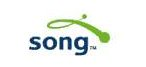# Song

SongOperatorformer
Address
CountryU.S.A.
Phone
Fax
Founded2003
Website
2-lettercodeDL
3-lettercodeDAL
CallsignDelta
Color scheme change
Remarkscompany operated last flight 30/04/2006; all aircraft went back to parent company Delta Air Lines
 historic fleet N608DA (c/n 22815) N609DL (c/n 22816) N610DL (c/n 22817) N613DL (c/n 22820) N614DL (c/n 22821) N615DL (c/n 22822) N638DL (c/n 23761) N639DL (c/n 23993) N640DL (c/n 23994) N641DL (c/n 23995) N643DL (c/n 23997) N644DL (c/n 23998) N645DL (c/n 24216) N647DL (c/n 24218) N648DL (c/n 24372) N649DL (c/n 24389) N650DL (c/n 24390) N651DL (c/n 24391) N652DL (c/n 24392) N654DL (c/n 24394) N655DL (c/n 24395) N684DA (c/n 27104) N685DA (c/n 27588) N693DL (c/n 29725) N694DL (c/n 29726) N695DL (c/n 29727) N696DL (c/n 29728) N697DL (c/n 30318) N698DL (c/n 29911) N699DL (c/n 29970) N6700 (c/n 30337) N6701 (c/n 30187) N6702 (c/n 30188) N6703D (c/n 30234) N6704Z (c/n 30396) N6705Y (c/n 30397) N6706Q (c/n 30422) N6707A (c/n 30395) N6708D (c/n 30480) N6709 (c/n 30481) N6710E (c/n 30482) N6711M (c/n 30483) N6712B (c/n 30484) N6713Y (c/n 30777) N6714Q (c/n 30485) N6715C (c/n 30486) N6716C (c/n 30838) N67171 (c/n 30839)